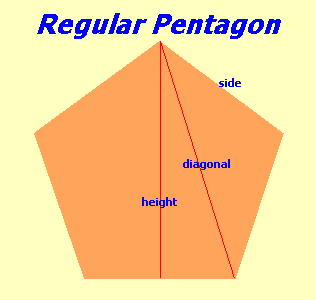# Area and Perimeter of a Pentagon

Calculate the area and perimeter of a regular pentagon shape. Includes the diagonal and the height length of the pentagon. In a regular pentagon all five sides are the same length and all five inside angles have the same size adding up to 540 degrees.### Area and Perimeter of a Pentagon

Select unit:
Side Length {{selectedunit.m1}}
decimal rounding # of digits in results

#### Results:

 Pentagon Area {{selectedunit.m1}}² Perimeter of Pentagon {{selectedunit.m1}} Diagonal {{selectedunit.m1}} Height {{selectedunit.m1}}

#### Calculation

1. enter the length of a side
2. number of decimal places required in results

#### Results

1. area of the pentagon
2. perimeter
3. diagonal
4. height